# Knowledge Base

### Welcome to OPAL-RT’s Knowledge Base

OPAL-RT offers a repository of support information for optimal use of its technology.

Please note that OPAL-RT knowledge base is not fully optimized for mobile platforms.

For optimal experience, use a desktop computer.

Reference Number: AA-02037 // Views: 22 // Created: 2021-09-16 07:36:42 // Last Updated: 2021-10-29 12:49:56
HowTo
How to simulate 3-single core transformer turn to turn fault in HYPERSIM

INTRODUCTION:

For a transformer that comprises of 3 single cores, the [z] based w/fault transformer blocks are not suitable to simulate its faults. The most proper way to simulate a winding-to-ground or winding-to-winding fault is using three 3-winding single phase transformers.

This KB explains the principle of simulate winding to ground and winding to winding faults for 3-single core transformer using 3-winding transformer blocks and how to set transformers’ corresponding parameters.

MODEL DESCRIPTION:

3-single-core transformer can be simply represented by three 1-ph transformers. The relationship between the terminal voltages and currents of the transformer can be expressed as matrix equation: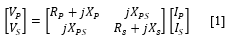where Vp , Vs and Ip , Is  are primary, secondary windings voltages and currents. Respectively, Rp , Rs , Xp , Xs ,  indicate the self-resistance and self-reactance in the primary and secondary winding; Xps is the mutual reactance between the primary and secondary winding.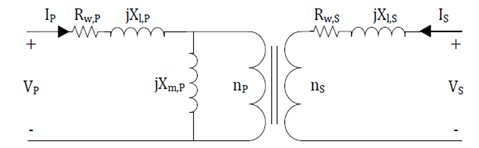Figure 1. Equivalent circuit of a single-phase transformer

Where Rw , Xl , Xm , np , and ns are winding resistance, leakage reactance, magnetizing reactance, turn number of primary winding and turn number of secondary winding, respectively.

The parameters' relationship of these two models can be described as: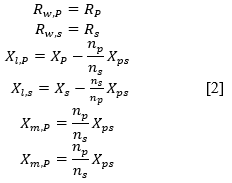GENERAL MODEL PRINCIPLE:

According to Figure1, the winding is divided into three sub-coils to model a TTF.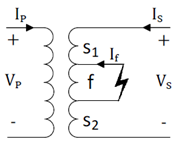Figure 2. A transformer with a TTF on its secondary winding

The transformer voltages and currents can be represent as matrix: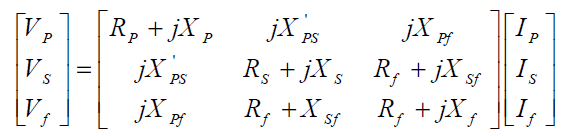using equation  to rewrite it, then it can be represent as an accurate equivalent circuit when the nf turns of secondary winding become shorted: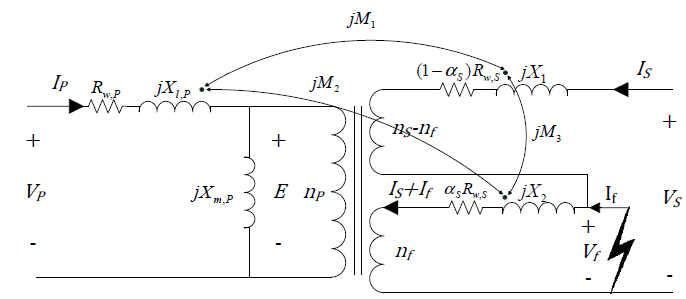Figure 3. Accurate equivalent circuit of a single phase transformer with a TTF on its secondary winding

Where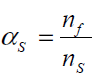For large transformers which have a primary winding tightly coupled to a secondary winding, it is a good approximation to assume that leakage flux between primary and secondary windings are equal to zero. The equations can be simplified to: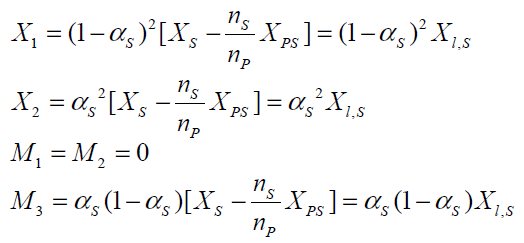Therefore, the simplified equivalent circuit of transformer, when the nf turns of the secondary winding were shorted, can be obtained as shown in figure 4.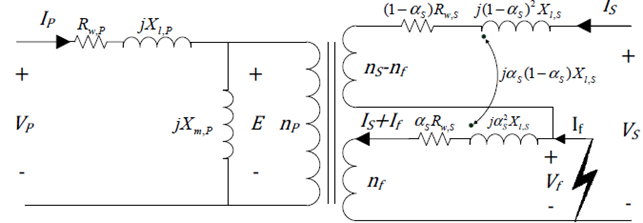Figure 4. Simplified equivalent circuit of a single phase transformer with a TTF on its secondary winding.

MODEL CREATION IN HYPERSIM:

The 3ph 2-winding transformer diagram in HYPERSIM block is as: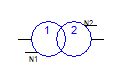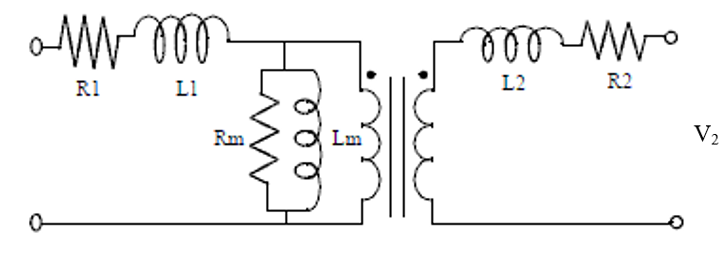Figure 5. Diagram of HYPERSIM block Linear 2-winding Transformer

Assume a nf turn to turn fault, i.e. a = nf/ns = 20% of the turn, happens in the secondary winding. It can be represented by 1-phase Transformer, 3-winding block (Figure 6) connected with a coupled R-L block (Figure 7) and leave the primary side parameters as it is.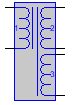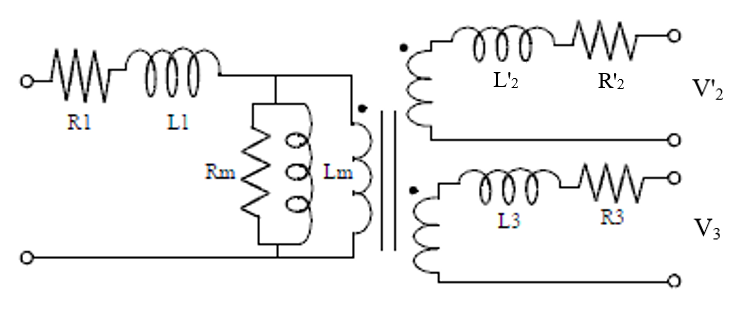Figure 6. Diagram of HYPERSIM block 1-phase Transformer, 3-winding

According to the derive process, the secondary winding parameters then split to 3 parts and their parameters can be calculated with the equations: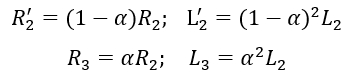Since the mutual inductance is simulated with a separate block, the voltage in the 1-ph 3 winding block then don't need to consider the affect caused by the mutual inductance. It makes the secondary V '2 and tertiary voltage Vfor 1ph 3 winding transformer has the relation with 2 winding transformer: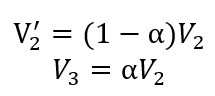And the mutual inductance for winding 2 and 3 present in the R-L coupled block is calculated by: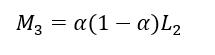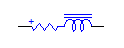Figure 7. HYPERSIM block coupled R-L

Then the Figure 4 equivalent model for 1-ph XFO with turn to turn on secondary side can be created in HYPERSIM as shown in Figure 8.

The key for the HYPERSIM model creation lays on the connection of Coupled R-L. The block itself is a three phase, here we use 2 channels (phases). To help understand the connection, corresponding Simulink model connection is shown in Figure 8 too.

Note: since the RL Coupled block is a 3-phase device, although one of the phase (i.e. phase c) is not used, it should be connected to ground and set a small self inductance value. Otherwise HYPERSIM topology calculation will have ill matrix.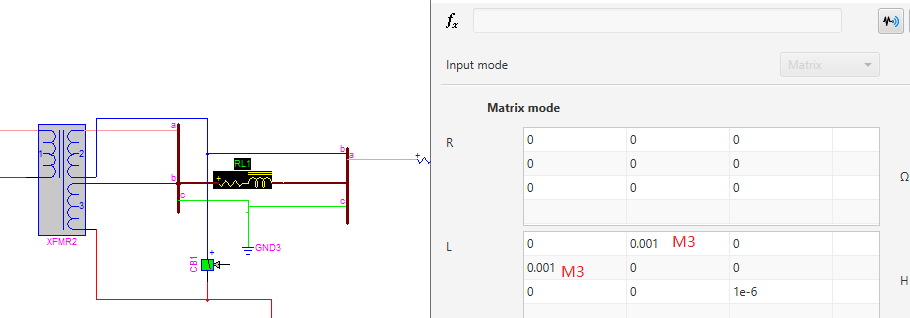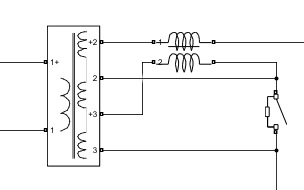Figure 8. HYPERSIM 1-phase model and Simulink model for transformer turn to turn fault

As an example, a 3-phase Yg/Yg connection in HYPERSIM then can be splited into 3 1-ph blocks with turn to turn fault connections as shown in Figure 9.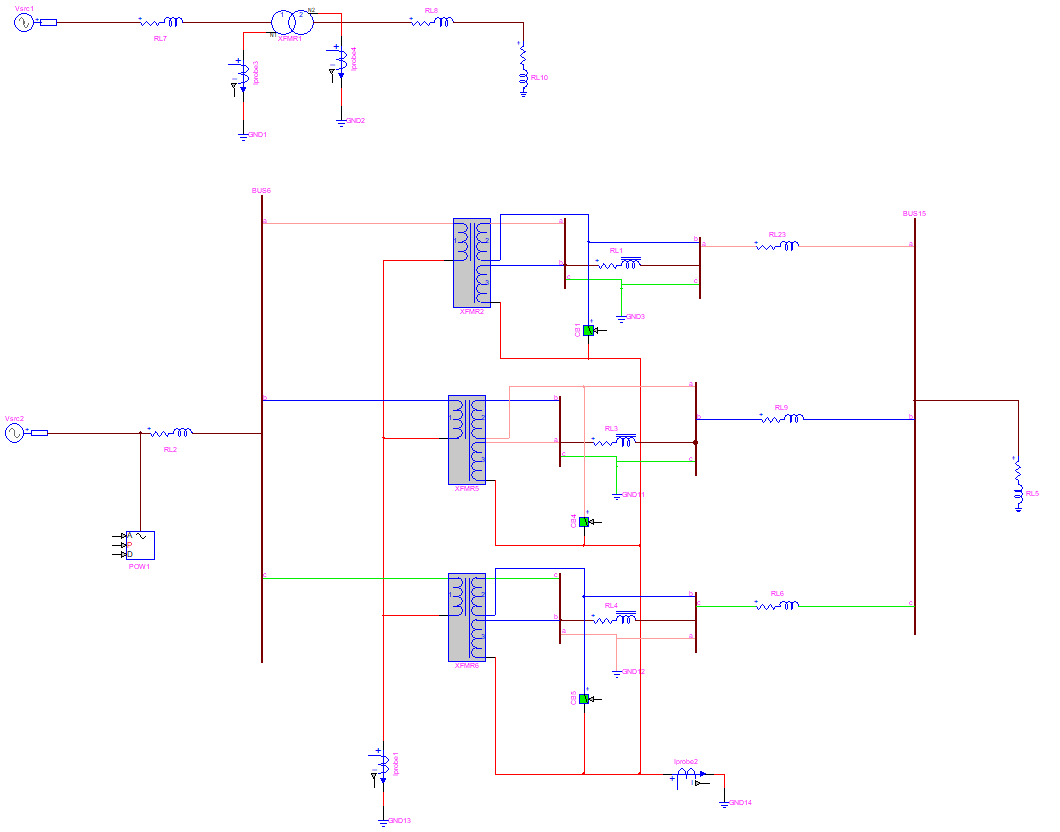Figure 9. HYPERSIM 3ph XFO model and 3 1-ph XFO model with turn to turn fault connections

The voltage and current on both side matches between the 3-ph block and 3 1-ph block models: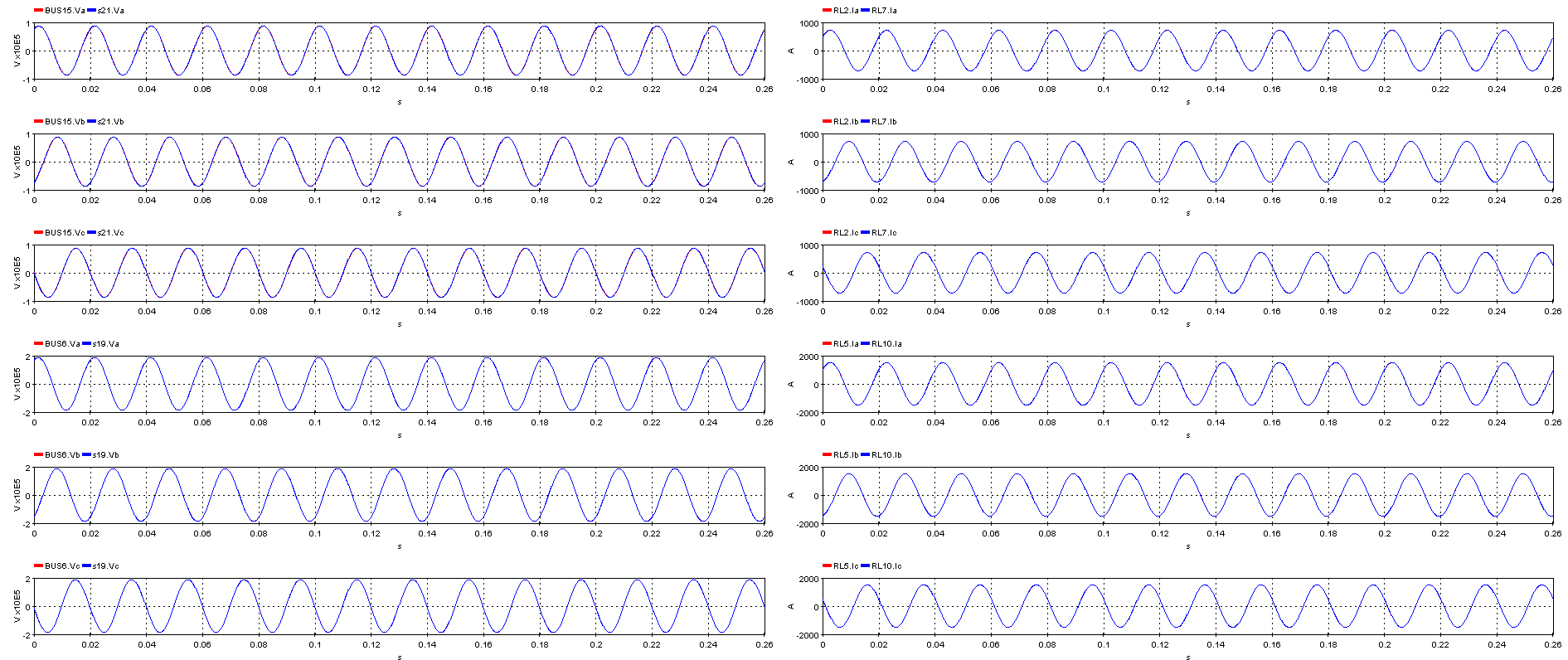Figure 10. HYPERSIM 3ph XFO model and 3 1-ph XFO model with turn to turn fault connections

EXAMPLE:

The same parameters are used in HYPERSIM model provided in the reference paper to simulate the TTF on secondary side and compare the result with, the test models are attached to the end of this KB.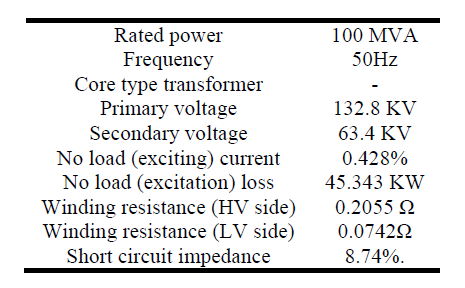Table 1. Example test parameters

the TTF fault on each phase at different time: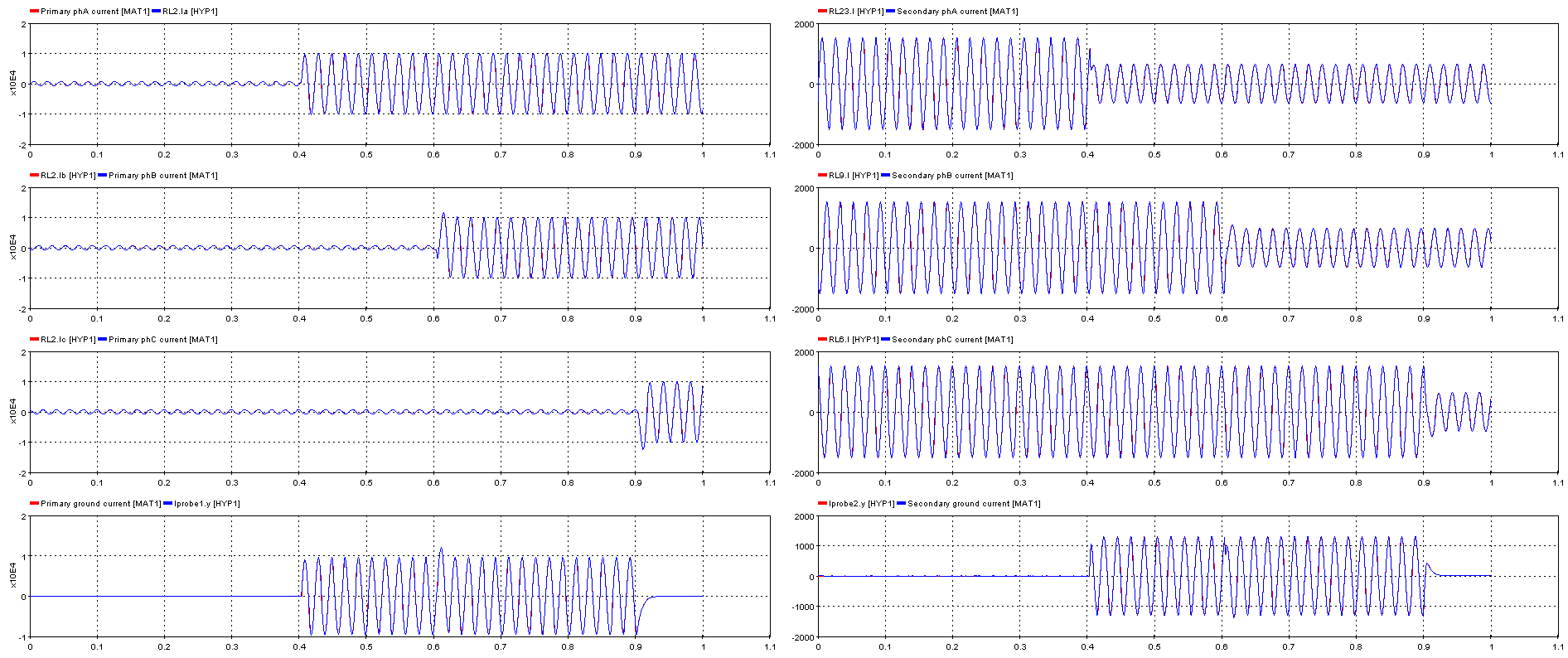Figure 11. Simulate TTF results compared with Matlab simPowerSystem model

 Gholami, Mohammad & Hajipour, Ehsan & Vakilian, Mehdi. (2016). A Single Phase Transformer Equivalent Circuit for Accurate Turn to Turn Fault Modeling. 10.1109/IranianCEE.2016.7585591.TTF.zip 1 Mb Download File